# 寻找市场形态的计量经济学方法：自相关，热点图和散点图

### 识别价格增量形态的经典计量经济学方法是自相关

```def standard_autocorrelation(symbol, lag):
rates = pd.DataFrame(MT5CopyRatesRange(symbol, MT5_TIMEFRAME_H1, datetime(2015, 1, 1), datetime(2020, 1, 1)),
columns=['time', 'open', 'low', 'high', 'close', 'tick_volume', 'spread', 'real_volume'])
rates = rates.drop(['open', 'low', 'high', 'tick_volume', 'spread', 'real_volume'], axis=1).set_index('time')
rates = rates.diff(lag).dropna()
from pandas.plotting import autocorrelation_plot
plt.figure(figsize=(10, 5))
autocorrelation_plot(rates)

standard_autocorrelation('EURUSD', 50)
```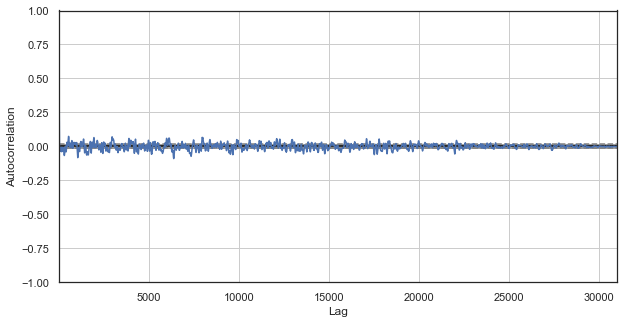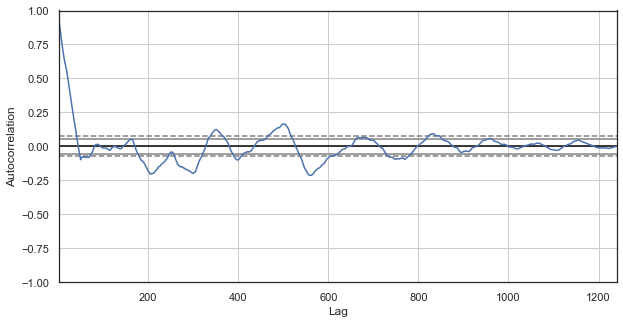### 在价格增量之中识别形态的自定义方法是季节性自相关

```def seasonal_autocorrelation(symbol, lag, hour1, hour2):
rates = pd.DataFrame(MT5CopyRatesRange(symbol, MT5_TIMEFRAME_H1, datetime(2015, 1, 1), datetime(2020, 1, 1)),
columns=['time', 'open', 'low', 'high', 'close', 'tick_volume', 'spread', 'real_volume'])
rates = rates.drop(['open', 'low', 'high', 'tick_volume', 'spread', 'real_volume'], axis=1).set_index('time')
rates = rates.drop(rates.index[~rates.index.hour.isin([hour1, hour2])]).diff(lag).dropna()
from pandas.plotting import autocorrelation_plot
plt.figure(figsize=(10, 5))
autocorrelation_plot(rates)

seasonal_autocorrelation('EURUSD', 50, 1, 2)
```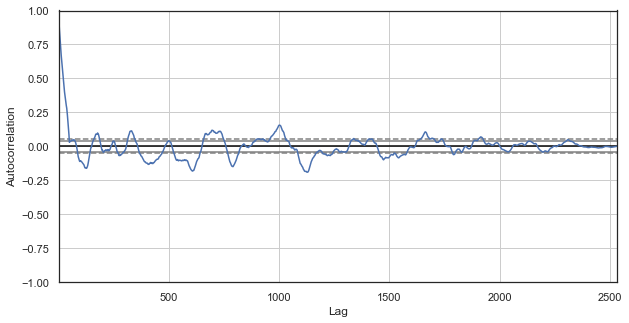### 所有时段的季节相关性热点图

```#calculate correlation heatmap between all hours
def correlation_heatmap(symbol, lag, corrthresh):
out = pd.DataFrame()
rates = pd.DataFrame(MT5CopyRatesRange(symbol, MT5_TIMEFRAME_H1, datetime(2015, 1, 1), datetime(2020, 1, 1)),
columns=['time', 'open', 'low', 'high', 'close', 'tick_volume', 'spread', 'real_volume'])
rates = rates.drop(['open', 'low', 'high', 'tick_volume', 'spread', 'real_volume'], axis=1).set_index('time')

for i in range(24):
ratesH = None
ratesH = rates.drop(rates.index[~rates.index.hour.isin([i])]).diff(lag).dropna()
out[str(i)] = ratesH['close'].reset_index(drop=True)
plt.figure(figsize=(10, 10))
corr = out.corr()
# Generate a mask for the upper triangle
return out

out = correlation_heatmap(symbol='EURUSD', lag=25, corrthresh=0.9)
```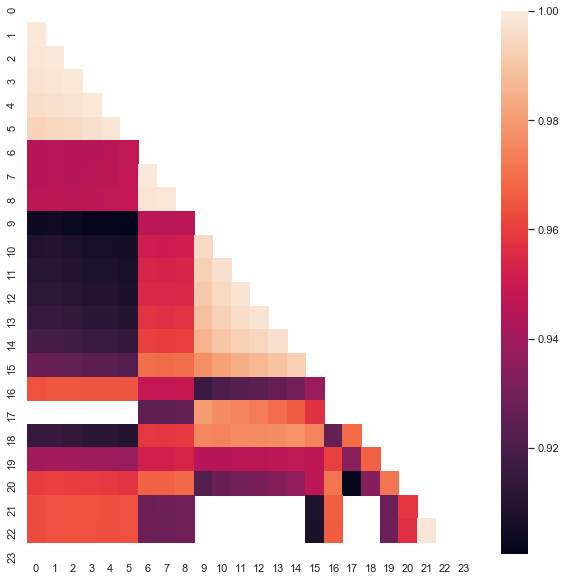`out[['10','11','12','13','14']].describe()`
10 11 12 13
count 1265.000000 1265.000000 1265.000000 1265.000000 1265.000000
mean -0.001016 -0.001015 -0.001005 -0.000992 -0.000999
std 0.024613 0.024640 0.024578 0.024578 0.024511
min -0.082850 -0.084550 -0.086880 -0.087510 -0.087350
25% -0.014970 -0.015160 -0.014660 -0.014850 -0.014820
50% -0.000900 -0.000860 -0.001210 -0.001350 -0.001280
75% 0.013460 0.013690 0.013760 0.014030 0.013690
max 0.082550 0.082920 0.085830 0.089030 0.086260

```plt.figure(figsize=(10,5))
plt.plot(out[['12','13','14']])
plt.legend(out[['12','13','14']])
plt.show()
```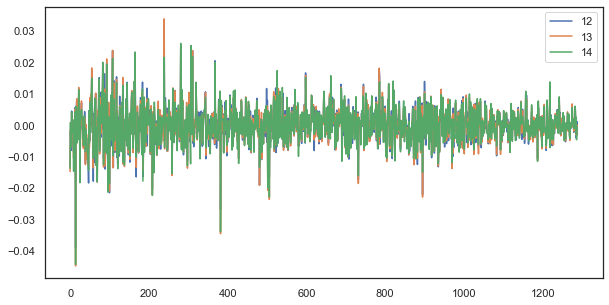### 规律性公式：简单但良好

```# calculate joinplot between real and predicted returns
def hourly_signals_statistics(symbol, lag,  hour, hour2, rfilter):
rates = pd.DataFrame(MT5CopyRatesRange(symbol, MT5_TIMEFRAME_H1, datetime(2015, 1, 1), datetime(2020, 1, 1)),
columns=['time', 'open', 'low', 'high', 'close', 'tick_volume', 'spread', 'real_volume'])
rates = rates.drop(['open', 'low', 'high', 'tick_volume', 'spread', 'real_volume'], axis=1).set_index('time')
# price differences for every hour series
H = rates.drop(rates.index[~rates.index.hour.isin([hour])]).reset_index(drop=True).diff(lag).dropna()
H2 = rates.drop(rates.index[~rates.index.hour.isin([hour2])]).reset_index(drop=True).diff(lag).dropna()
# current returns for both hours
HF = H[1:].reset_index(drop=True); HL = H2[1:].reset_index(drop=True)
# previous returns for both hours
HF2 = H[:-1].reset_index(drop=True); HL2 = H2[:-1].reset_index(drop=True)

# Basic equation:  ret[-1] = ret - (ret[lag] - ret[lag-1])
# or Close[-1] = (Close-Close[lag]) - ((Close[lag]-Close[lag*2]) - (Close[lag-1]-Close[lag*2-1]))

predicted = HF-(HF2-HL2)
real = HL
# correlation joinplot between two series
outcorr = pd.DataFrame()
outcorr['Hour ' + str(hour)] = H['close']
outcorr['Hour ' + str(hour2)] = H2['close']
# real VS predicted prices
out = pd.DataFrame()
out['real'] = real['close']
out['predicted'] = predicted['close']
out = out.loc[((out['predicted'] >= rfilter) | (out['predicted'] <=- rfilter))]
# plptting results
from scipy import stats
sns.jointplot(x='Hour ' + str(hour), y='Hour ' + str(hour2), data=outcorr, kind="reg", height=7, ratio=6).annotate(stats.pearsonr)
sns.jointplot(x='real', y='predicted', data=out, kind="reg", height=7, ratio=6).annotate(stats.pearsonr)

hourly_signals_statistics('EURUSD', lag=25, hour=13, hour2=14, rfilter=0.00)
```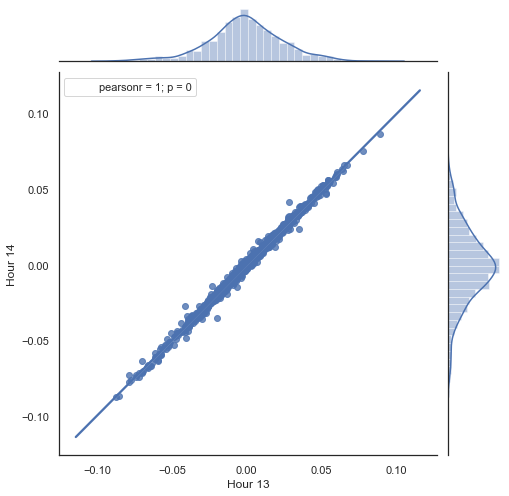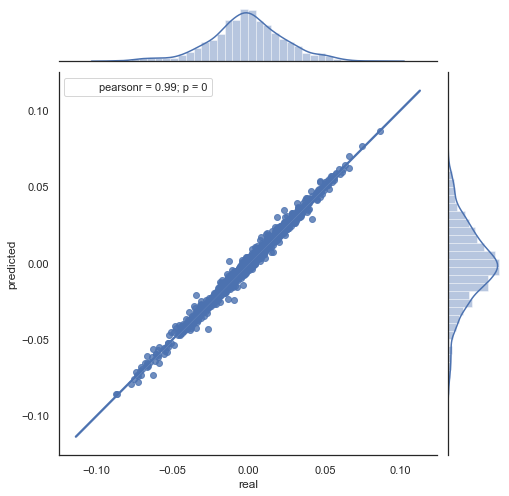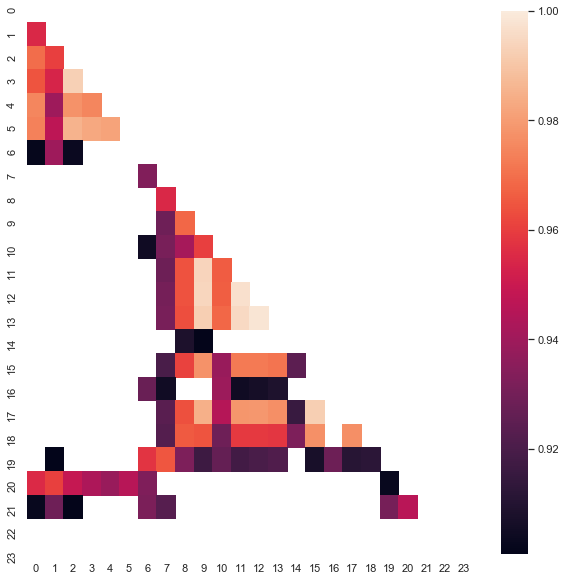10 11 12 13
count 5151.000000 5151.000000 5151.000000 5151.000000 5151.000000
mean 0.000470 0.000470 0.000472 0.000472 0.000478
std 0.037784 0.037774 0.037732 0.037693 0.037699
min -0.221500 -0.227600 -0.222600 -0.221100 -0.216100
25% -0.020500 -0.020705 -0.020800 -0.020655 -0.020600
50% 0.000100 0.000100 0.000150 0.000100 0.000250
75% 0.023500 0.023215 0.023500 0.023570 0.023420
max 0.213700 0.212200 0.210700 0.212600 0.208800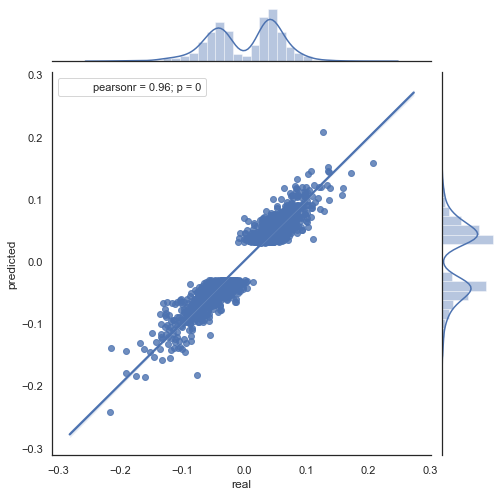```# calculate joinplot between real an predicted returns
def hourly_signals_statistics3D(symbol, lag,  hour, hour2, rfilter):
rates = pd.DataFrame(MT5CopyRatesRange(symbol, MT5_TIMEFRAME_H1, datetime(2015, 1, 1), datetime(2020, 1, 1)),
columns=['time', 'open', 'low', 'high', 'close', 'tick_volume', 'spread', 'real_volume'])
rates = rates.drop(['open', 'low', 'high', 'tick_volume', 'spread', 'real_volume'], axis=1).set_index('time')
rates = pd.DataFrame(rates['close'].diff(lag)).dropna()

out = pd.DataFrame();
for i in range(hour, hour2):
H = None; H2 = None; HF = None; HL = None; HF2 = None; HL2 = None; predicted = None; real = None;
H = rates.drop(rates.index[~rates.index.hour.isin([hour])]).reset_index(drop=True)
H2 = rates.drop(rates.index[~rates.index.hour.isin([i+1])]).reset_index(drop=True)
HF = H[1:].reset_index(drop=True); HL = H2[1:].reset_index(drop=True);     # current hours
HF2 = H[:-1].reset_index(drop=True); HL2 = H2[:-1].reset_index(drop=True)  # last day hours

predicted = HF-(HF2-HL2)
real = HL

out3D = pd.DataFrame()
out3D['real'] = real['close']
out3D['predicted'] = predicted['close']
out3D['predictedABS'] = predicted['close'].abs()
out3D['hour'] = i
out3D = out3D.loc[((out3D['predicted'] >= rfilter) | (out3D['predicted'] <=- rfilter))]

out = out.append(out3D)

import plotly.express as px
fig = px.scatter_3d(out, x='hour', y='predicted', z='real', size='predictedABS', color='hour', height=1000, width=1000)
fig.show()

hourly_signals_statistics3D('EURUSD', lag=24, hour=10, hour2=23, rfilter=0.000)
```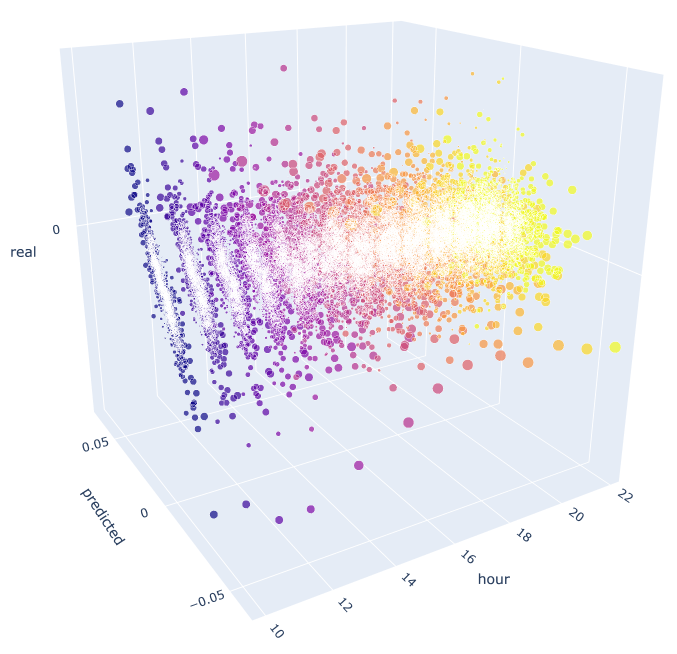### 依据识别出的季节性形态进行交易的智能交易系统示例

```input int OpenThreshold = 30;     //Open threshold
input int OpenThreshold1 = 30;    //Open threshold 1
input int OpenThreshold2 = 30;    //Open threshold 2
input int OpenThreshold3 = 30;    //Open threshold 3
input int OpenThreshold4 = 30;    //Open threshold 4
input int Lag = 10;
input int stoploss = 150;         //Stop loss
input int OrderMagic = 666;       //Orders magic
input double   MaximumRisk=0.01;  //Maximum risk
input double   CustomLot=0;       //Custom lot
```

```void OnTick() {
//---
if(!isNewBar()) return;
CopyClose(NULL, 0, 0, Lag*2+1, prArr);
ArraySetAsSeries(prArr, true);
const double pr = (prArr - prArr[Lag]) - ((prArr[Lag] - prArr[Lag*2]) - (prArr[Lag-1] - prArr[Lag*2-1]));

TimeToStruct(TimeCurrent(), hours);

if(hours.hour >=10 && hours.hour <=14) {

//if(countOrders(0)==0)

if(pr <= -signal && hours.hour==10)
if(pr <= -signal1 && hours.hour==11)
if(pr <= -signal2 && hours.hour==12)
if(pr <= -signal3 && hours.hour==13)
if(pr <= -signal4 && hours.hour==14)
}
}
}
```

“pr” 常数由上述指定公式计算得出。 该公式预测下一根柱线的价格增加。 然后检查每个时段的条件。 如果增量达到特定时段的最小阈值，则达成卖出。 我们已经发现，在 2015 年至 2020 年间，若平均增量转移到负数区域会令买入无效，您可自行检查这一点。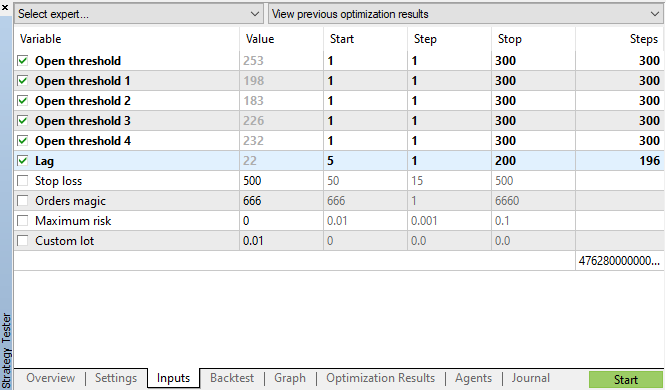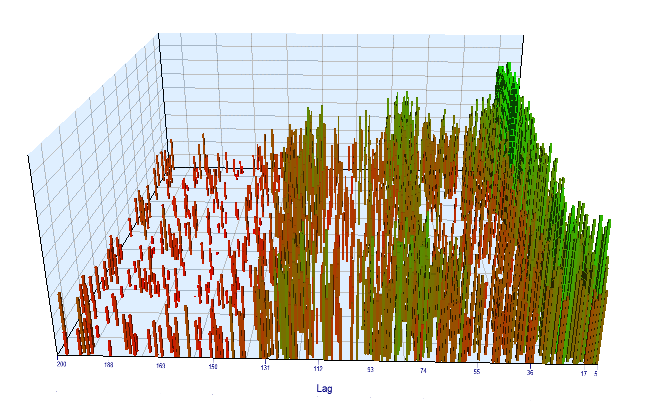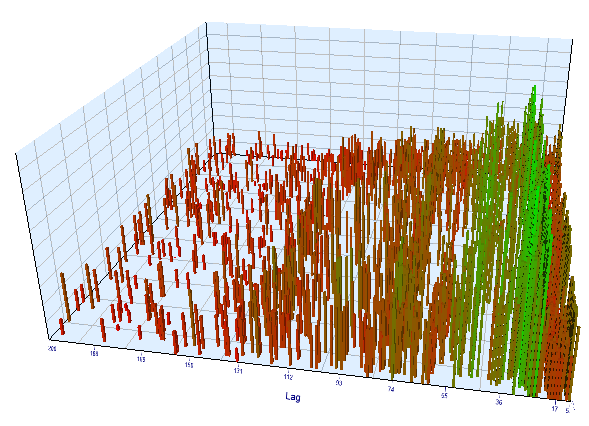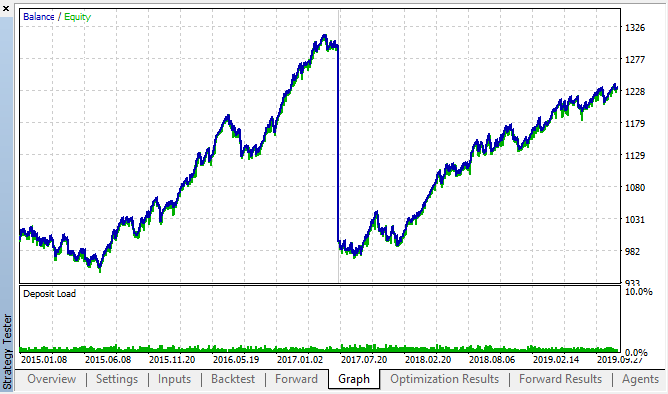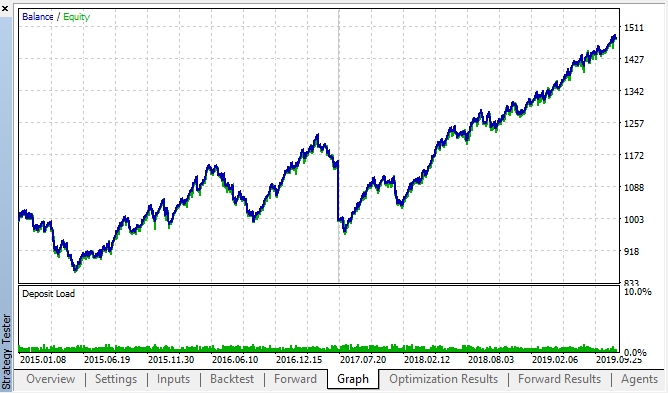### 检查另一个时间帧的增量周期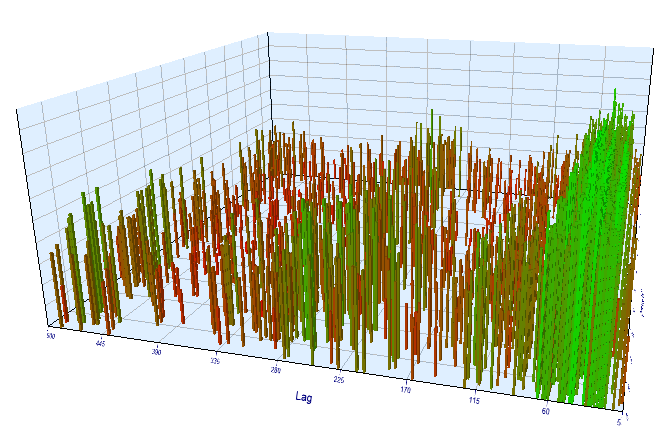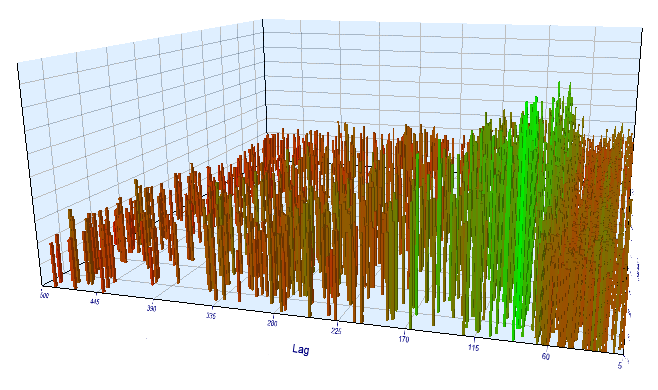## 量化课程

移动端课程